# Geometric Statistics

The Geometric Statistics module adds a couple of image statistic variables centered around image geometry. These variables can then be used elsewhere in RoboRealm, for example in the conditional statement, VBScript module or exported to external programs using RoboRealm Plugins.

## Interface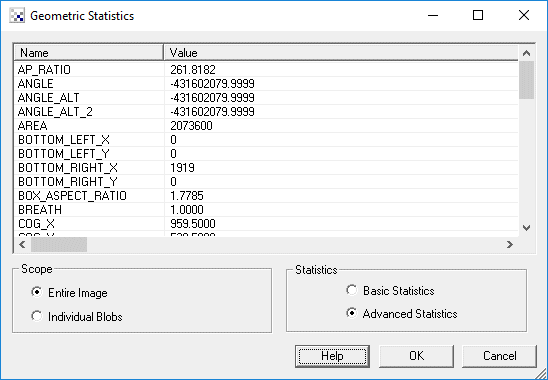1. Scope - Click on the appropriate radio button to select if you want the statistics to be generated from the entire image or from an individual blob. If you select individual blobs non-black pixels in the current image will be used to form blobs. To see the statistics for an individual blob click on the blob within the main RoboRealm GUI window. That will switch the variable(x) denotation to that particular blob.

2. Statistics - The module can produce many statistical numbers based on the current image. To reduce CPU load for those applications that only require some basic numbers you can select Basic Statistics that will produce a subset of Advanced Statistics that are faster to compute.

## Variables

AREA - the number of non-black (0,0,0) pixels in the current image

ANGLE - the orientation angle of the blob. This is calculated by determining the angle from the center of gravity of the blob to the furthest point from that center along the blob's perimiter.

ANGLE_ALT - alternative orientation angle of the blob. This is calculated by determining the vector formed by the center of gravity to the perimiter's center of gravity. If your blob has holes in it this may be a more stable way to determine blob orientation than ANGLE.

ANGLE_ALT_2 - alternative orientation angle of the blob. This is calculated by determining the average vector formed from a current perimiter point to the center of gravity.

COG_X, COG_Y - the center of gravity of the blob

PERIMETER - the number of pixels that surround non black blobs

AP_RATIO - AREA / PERIMETER, determines how round an image is; also known as "Perimeter Equivalent Diameter".

EQUIV_DIAMETER - the diameter of a circle with the same area as the region. Calculated as sqrt(4*Area/pi)

COMPACTNESS - (PERIMETER*PERIMETER) / AREA : ratio of the square of the perimeter to the area; also known as "shape".

COMPACTNESS_2 - sqrt(4*AREA/PI) / FERET : alternate compactness measure

CIRCULARITY - 4*PI*AREA/(PERIMETER*PERIMETER), measure of circularity.

CIRCULARITY_2 - alternate measure of circularity.

FERET - Feret's diameter also known as the caliper length or largest axis length: the greatest distance between any two non-zero pixels

FERET_AREA - FERET * BREATH; also know as Feret's bounding box

FERET_ORIENTATION - orientation of the Feret's diameter in degrees

FERET_FIRST_X
FERET_FIRST_Y
FERET_SECOND_X
FERET_SECOND_Y
- points that makeup the Feret diameter

BREATH - the largest axis perpendicular to the Feret diameter

ROUNDNESS - 4*AREA/(Pi*(FERET*FERET)), measure of roundness

MIN_X - minimum bounding box x coordinate

MIN_Y - minimum bounding box y coordinate

MAX_X - maximum bounding box x coordinate

MAX_Y - maximum bounding box y coordinate

EXTENT - proportion of the pixels in the bounding box that are also in the region. Calculated as (blob area / bounding box area).

TOP_LEFT_X
TOP_LEFT_Y
TOP_RIGHT_X
TOP_RIGHT_Y
RIGHT_TOP_X
RIGHT_TOP_Y
RIGHT_BOTTOM_X
RIGHT_BOTTOM_Y
BOTTOM_LEFT_X
BOTTOM_LEFT_Y
BOTTOM_RIGHT_X
BOTTOM_RIGHT_Y
LEFT_TOP_X
LEFT_TOP_Y
LEFT_BOTTOM_X
LEFT_BOTTOM_Y
- the extrema points of the image

FERET_ASPECT_RATIO - FERET/BREATH

BOX_ASPECT_RATIO - (MAX_X-MIN_X)/(MAX_Y-MIN_Y)

WIDTH - the width of the object in pixels

HEIGHT - the height of the object in pixels

Note that you can highlight the appropriate statistics and press CTRL-C to copy the information to your clipboard. This information can then be pasted into Excel or other statistics programs.

 Geometric_Statistics Related Forum Posts Last post Posts ViewsArea of Multiple Blobs Hi there, Thanks for the great program you have created! I really appreciate the effort put into it. I would like ... 6 years 6 1699 Multiple Geometric Statistics module Hi, I am trying to perform two sets of geometric analyses in the same pipeline, but am not sure how... 7 years 5 1713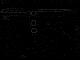Area of region I'm try to use RR to calculate the area of two round, but not exactly circular, regions in an image. By using edge dection (as ... 7 years 5 1974 BREATH variable Hi STeven, I have noticed that the BREATH variable does not appear in the Watch_Variables module. I... 7 years 2 1766 creating a bounding box over a blob group Hi STeven, Is there a way to place a bounding box over a group of blobs?... 8 years 3 1882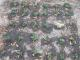Multi object tracking and triggering Here is the working vbscript It work amazingly great for multiblob tracking and triggering. I found very tricky to work with it.... 8 years 12 3679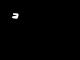Tracking a \"U\" shape of lights I have a rectangular robot with LED strips around three edges. I'm successfully using RoboRealm to isolate the light from the r... 10 years 5 2592Blob Segmentation and Filtering : Human Targets Hi, I have been using the movement module to track a single target. It is now my goal to track more than 1 target, starting firs... 10 years 11 4281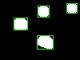two boxes around two different coloured balls hi there i would like to have two different boxes around two different coloured balls so i would l... 10 years 2 2610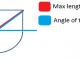Detecting angle of blob Is this possible, I�m new to roborealm. i want a to detect a blob like see the attachment. When viewing the object... 11 years 6 4250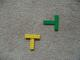Variable Values on VBScript Hello, I am doing a visual odometry for 3 robots with a camera (determinate the X,Y,theta - positio... 11 years 14 5705 COLOUR_STATISTICS averages I am trying to do fine colour comparisons of items presented to a camera. I am using the COLOUR_STATISTICS after t... 12 years 2 2609Locate brightest pixel I'm trying to use roborealm to locate the brightest pixel. Does this feature already exist? Thanks!... 12 years 2 2812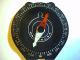Compass reading Howdy= Im a beginner and could use a little help. I would like to read the compass heading from a compass. The camera and compas... 12 years 4 2938Geometric Statistics Problem Hi Steven and Prof Mason,     Thanks for ur help in my last post about marker p... 12 years 3 3069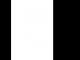Image Module Problem Hello Steven, [image1] [image2] I�m trying to align/regi... 12 years 9 3815SRV- 1B Hi. I am using the SRV - 1B for my project and I need to detection of visual markers. The deal with the visual markers is each o... 12 years 2 2992triangle direction hi! how can i find a triangle direction using RR? ... 12 years 5 4065